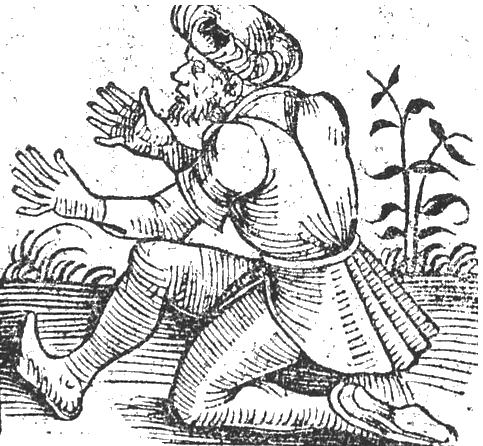### Carry On

September 8, 2010

Imagine for a moment that you're a young child again, and you're just being taught how to add large numbers. Sure, you can do 5 + 3 = 8, but now they hit you with 5 + 6 = ?. You have just ten fingers, so counting on your fingers doesn't work, and the teacher might object to your taking your shoes off. Then they teach you about carrying digits, so you can compute 5 + 6 = 11, just like the big boys do. How simpler life would be if you didn't need to carry!

Well, that's the idea that Marc LeBrun of Fixpoint Inc.,Novato, CA, and David Applegate and Neil J. A. Sloane of AT&T Shannon Labs decided to investigate. Neil J. A. Sloane may be known to many of you as the originator of the The On-Line Encyclopedia of Integer Sequences, something he started on index cards while a graduate student at Cornell University in 1965. These integer sequences have gone through publication twice in book form, finally developing a life of their own on the internet with 175,000 entries. If still printed in book form, the integer sequences would fill 750 volumes; and these volumes would not be very useful when it came to searching for your particular sequence.

In mathspeak, Addition and multiplication of single-digit numbers in a carryless arithmetic system are performed "reduction mod 10, meaning simply that the carry digits are ignored. Take, for example, this simple addition
7 8 5
+3 7 6
0 5 1
and this simple multiplication
6 4 3
x    5 9
4 6 7
0 0 5
0 4 1 7
You get the idea. The authors investigated the manifold consequences (pun intended) of this modulus type of arithmetic. We'll just summarize the idea of prime numbers. First, there can be no prime numbers in the usual sense, since all numbers in the carryless arithmetic system are divisible by nine. Here's the logic behind that
1. Note that 9 = 9 x 1, 8 = 9 x 2, 7 = 9 x 3, etc.
2. This means we can replace all nines in a number by nine time one, all eights in a numbers by nine times two, etc.
3. But every digit in the number is now known to be divisible by nine
4. So, the number is divisible by nine.
Not having primes is no fun, especially for number theorists, so they redefine the concept of a prime number as follows. Noting that 1 x 1 = 1, 3 x 7 = 1, and 9 x 9 = 1, they call numbers like 1, 3, 7 and 9 the "units." So they define a prime in the carryless arithmetic system to be those numbers that are only the product of a unit and itself. Eleven, a prime in the usual number system, is not a prime in the carryless system, since 11 = 51 x 61. The authors did some computer experiments that indicate that the first few carryless primes are 21, 23, 25, 27, 29, 41, 43, 45, 47, 49, 51, 52, 53, 54, 56, 57, 58, 59, 61, 63, . . . . Noting that 2 = 2 x 5005505551, the authors were worried that 21 might be the product of two huge numbers, and so on, for all of their carryless primes. Some fancy mathematics (better explained in Ref. ), allowed them to generate a list of assured carryless primes that's now integer sequence A169887.Advocate of a base-twelve number system. Illustration from the Nuremberg Chronicle by Hartmann Schedel (1440-1514).

### Reference:

Linked Keywords: Decimal; Novato, CA; Neil J. A. Sloane; AT&T Shannon Labs; The On-Line Encyclopedia of Integer Sequences; Cornell University; modular arithmetic; manifold; prime numbers; number theorists; integer sequence A169887; twelve fingers.